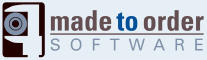# libsswf: sswf::Edges::edge_t Class Reference

The basic structure used to hold the coordinates of an edge. More...

`#include <libsswf.h>`

## Public Member Functions

edge_t (const edge_t &edge)
Copy an edge structure.
edge_t (long x, long y, long ctrl_x, long ctrl_y)
Constructs a curve edge structure.
edge_t (long x, long y)
Constructs a line edge structure.
edge_t (void)
Constructs a default edge structure.
bool IsLine (void) const
Check whether the edge represents a line (true) or a curve (false).
edge_toperator= (const edge_t &edge)
Copy an edge structure.

## Public Attributes

long f_ctrl_x
The control X coordinate or LONG_MIN.
long f_ctrl_y
The control Y coordinate or LONG_MIN.
long f_x
The X coordinate.
long f_y
The Y coordinate.

## Detailed Description

This helper structure is used to hold edges internally. You can also use it to pass edges to an Edges object.

The points are all specified as two dimension coordinates in TWIPS (1/20th of a pixel.)

Multiple points are specified as cumulative which means that the next point coordinates are added to the previous points coordinates. Thus a starting edge at (3, 7), followed by an edge at (9, 11) represents one point at (3, 7) and another at (12, 18).

Do not forget that this applies to control points as well. In an edge_t structure you first move to the (x, y) coordinate, and then to the (ctrl_x, ctrl_y) coordinates.

Note:
The fact that this structure is called Edges instead of Points is because of this fact. Edges coordinates are cumulative and not direct points. However, I most of the time will reference edges as points and vice versa.

For those of you who have played with a turtle under Logo, you can view this technique as if you were moving a turtle. It is very simiar (except that you do not have a direction since you move with relative coordinates.)

``` ADVANCE [x1, y1]
...
```

Warning:
This sub-structure is not currently accessible to the C library because it includes functions.
sswf::Edges

## Constructor & Destructor Documentation

 sswf::Edges::edge_t::edge_t ( void ) ` [inline]`

The default constructor creates a line edge at coordinate (0, 0).

References f_ctrl_x, f_ctrl_y, f_x, and f_y.

 sswf::Edges::edge_t::edge_t ( long x, long y ) ` [inline]`

This constructor creates a line edge at coordinate (x, y).

Parameters:
 x The horizontal coordinate y The vertical coordinate

References f_ctrl_x, f_ctrl_y, f_x, and f_y.

 sswf::Edges::edge_t::edge_t ( long x, long y, long ctrl_x, long ctrl_y ) ` [inline]`

This constructor creates a curve edge at coordinate (x, y) and control point (ctrl_x, ctrl_y).

Parameters:
 x The horizontal coordinate y The vertical coordinate ctrl_x The horizontal control coordinate ctrl_y The vertical control coordinate

References f_ctrl_x, f_ctrl_y, f_x, and f_y.

 sswf::Edges::edge_t::edge_t ( const edge_t & edge ) ` [inline]`

The copy operator copies the input edge in 'this' edge.

Note:
It should not be necessary to make this copy operator explicit, but some older compilers have a hard time without it.
Parameters:
 edge The edge to copy in 'this' edge_t

References f_ctrl_x, f_ctrl_y, f_x, and f_y.

## Member Function Documentation

 sswf::Edges::edge_t::IsLine ( void ) const` [inline]`

This function returns true if one of the control point is set to LONG_MIN. You should use that function and not try to test yourself whether an edge represents a line or a curve.

Returns:
true if the edge represents a line, false when it represents a curve

References f_ctrl_x, and f_ctrl_y.

Referenced by sswf::Edges::SaveEdge().

 sswf::Edges::edge_t::operator= ( const edge_t & edge ) ` [inline]`

The copy operator copies the input edge in 'this' edge.

Note:
It should not be necessary to make this copy operator explicit, but some older compilers have a hard time without it.
Parameters:
 edge The edge to copy in 'this'
Returns:
'this' edge which is now a copy of the input edge

References f_ctrl_x, f_ctrl_y, f_x, and f_y.

## Member Data Documentation

This variable member represents the Control point horizontal coordinate.

When set to LONG_MIN, it is ignored—no control point meaning that the edge represents a line.

This value is in TWIPS.

Referenced by edge_t(), IsLine(), operator=(), and sswf::Edges::SaveEdge().

This variable member represents the Control point vertical coordinate.

When set to LONG_MIN, it is ignored--no control point meaning that the edge represents a line.

Referenced by edge_t(), IsLine(), operator=(), and sswf::Edges::SaveEdge().

This variable member represents the horizontal coordinate of this edge.

This value is in TWIPS.

Referenced by edge_t(), operator=(), and sswf::Edges::SaveEdge().

This variable member represents the vertical coordinate of this edge.

This value is in TWIPS.

Referenced by edge_t(), operator=(), and sswf::Edges::SaveEdge().

The documentation for this class was generated from the following files:

Generated on Wed Mar 18 15:13:58 2009 for libsswf by1.5.5